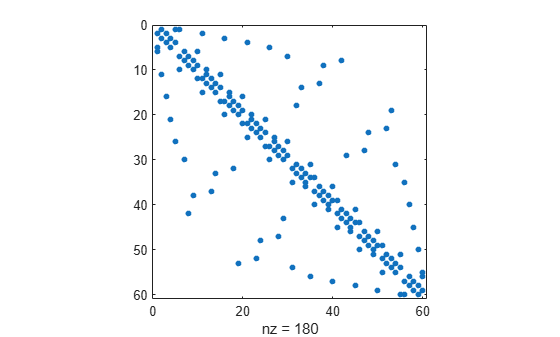sprand

Sparse uniformly distributed random matrix

Description

example

R = sprand(S) creates a sparse matrix that has the same sparsity pattern as the matrix S, but with uniformly distributed random entries.

example

R = sprand(m,n,density) creates a random m-by-n sparse matrix with approximately density*m*n uniformly distributed nonzero entries for density in the interval [0,1].

example

R = sprand(m,n,density,rc) creates a matrix that also has reciprocal condition number approximately equal to rc. The matrix R is constructed from a sum of matrices of rank one.

Examples

collapse all

Create the 60-by-60 sparse adjacency matrix of the connectivity graph of the Buckminster Fuller geodesic dome. Plot the sparsity pattern of the matrix S.

S = bucky;
spy(S)Create another sparse matrix R that has the same sparsity pattern as the matrix S, but with uniformly distributed random entries. Plot the sparsity pattern of R.

R = sprand(S);
spy(R)Create a random 500-by-1000 sparse matrix with density 0.1. The matrix has approximately 0.1*500*1000 = 50000 uniformly distributed nonzero entries.

R = sprand(500,1000,0.1);

Show the exact number of nonzero elements in the matrix R.

n = nnz(R)
n = 47663

Create a random 50-by-100 sparse matrix with approximately 0.2*50*100 = 1000 uniformly distributed nonzero entries. Specify the reciprocal condition number of the matrix to be 0.25.

R = sprand(50,100,0.2,0.25);

Show that the condition number of the matrix R is equal to 1/0.25 = 4.

cond(full(R))
ans = 4.0000

Input Arguments

collapse all

Input matrix, specified as a full or sparse matrix.

Data Types: single | double | int8 | int16 | int32 | int64 | uint8 | uint16 | uint32 | uint64 | logical
Complex Number Support: Yes

Number of matrix rows, specified as a nonnegative integer.

Number of matrix columns, specified as a nonnegative integer.

Density of nonzero elements, specified as a scalar. density must be in the interval [0,1].

Data Types: double

Reciprocal condition number, specified as a scalar or vector. rc must be in the interval [0,1].

If rc is a vector of length lr, where lr <= min(m,n), then R = sprand(m,n,density,rc) has rc as its first lr singular values and all others are zero. In this case, R is generated by random plane rotations applied to a diagonal matrix with the given singular values. It has a great deal of topological and algebraic structure.

Data Types: double

Limitations

• sprand is designed to produce large matrices with small density and will generate significantly fewer nonzero values than requested if m*n is small or density is large.

Tips

• sprand uses the same random number generator as the rand, randi, and randn functions. You can control this generator with the rng function.